Related Articles
Excel VBA | Average() Functions
• Last Updated : 03 Jul, 2018

VBA (Visual Basic for Applications) is the programming language of Excel and other offices. It is an event-driven programming language from Microsoft.

With Excel VBA, one can automate many tasks in excel and all other office softwares. It helps in generating reports, preparing various charts, graphs and moreover, it performs calculation using its various functions.
Let’s see Average functions in Excel.

1. AVERAGE It returns the arithmetic mean of all of its arguments.
Syntax:
`=AVERAGE(number1, number2, …)`

Here,
number 1: This is the first number in your cell. You can specify it upto 255 numbers.
number 2: This is an Optional field. You can specify it upto 255 numbers.

Example: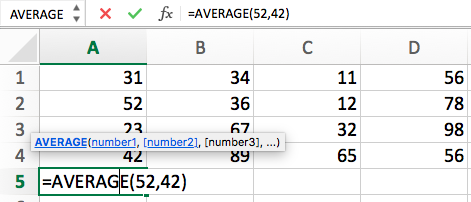Output: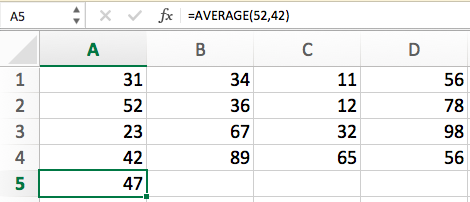2. AVERAGEIF: It calculates the average of only those values which meet a certain criteria.

Syntax:

`=AVERAGEIF(range, criteria, average_range)`

range: It is the range of cells to be evaluated. The cells in range can be numbers, names, arrays or any other reference that contains numbers. Blank and text values are not considered.

criteria: It should be in the form of a number or any expression which defines which all cells to be averaged. For instance, “mango”, C6, “<35”

average_range: [Optional] If this is not mentioned, Excel will get average of only those cells on which criteria is applied, which are specified in the range argument.

Notes:
-> If a cell in average_range is an empty cell, it will be ignored.
-> If range is a text value or blank, it will return an error.
-> If a cell in criteria is empty, it will be considered as 0 value.
-> If no cell meets the criteria, it will return an error.

Example: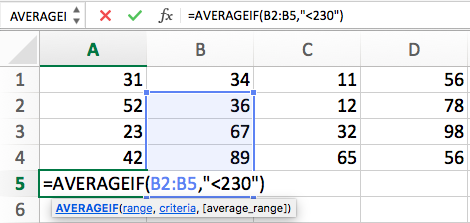Output: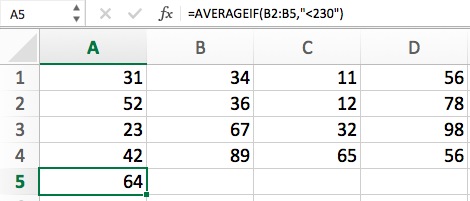3. AVERAGEIFS: Calculate average of cells that meet multiple criteria.
Syntax:
`=AVERAGEIFS(average_range, criteria_range1, criteria1, [criteria_range2, criteria2], …)`

average_range: (Required) One or more cells to calculate average.

criteria_range1: (Required) 1 to 127 ranges to evaluate the given criteria.

criteria1: 1 to 127 criteria in the form of numbers, expression to be averaged. This defines which all cells in criteria_range1 are to be averaged. For instance, criteria can be like this, “>32”, “apple”, “D7”

Example: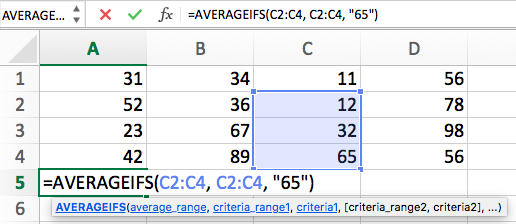Output: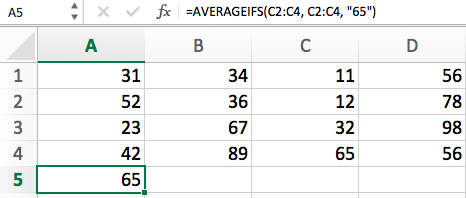My Personal Notes arrow_drop_up